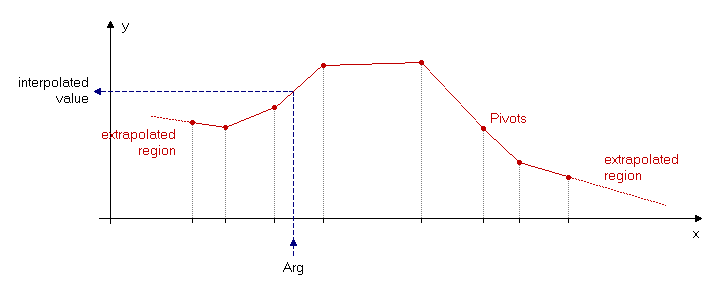The SDL Component Suite is an industry leading collection of components supporting scientific and engineering computing. Please visit the SDL Web site for more information....## InterpolXYTable

 Unit: SDL_math1 Class: None Declaration: function InterpolXYTable (Arg: double; const XYPivots: TDouble2DArray): double;

The function InterpolXYTable interpolates a function defined by the open array of irregularly spaced but sorted pivot points (parameter XYPivots) at the x position given by Arg. The sort order of the pivot points does not matter. The x and y coordinates of the pivot points are defined by the two-dimensional array XYPivots. The first column of the array contains the x values, the second column the y values. Each row of the array specifies a pivot point. If the argument Arg is less than the lowest x value (i.e. XYPivots[0,0]) or greater than the highest x value (XYPivots[0,length(XYPivots)]) the returned function value is extrapolated using the first or the last line segment, respectively.

The function raises an exception if the pivot table contains less than two pivot elements or if the number of columns is not equal to two.Last Update: 2020-Aug-14